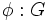# Difference between revisions of "Homomorphism of groups"

VIEW RELATED: Analogues of this | Variations of this | Opposites of this |[SHOW MORE]

Homomorphism redirect here. For the more general definition of homomorphism, refer homomorphism of universal algebras

## Definition

### Textbook definition (with symbols)

Let$G$ and$H$ be groups. Then a map$\phi: G$$H$ is termed a homomorphism of groups if$\phi$ satisfies the following condition:$\phi(ab) = \phi(a) \phi(b)$ for all$a, b$ in$G$

### Universal algebraic definition (with symbols)

Let$G$ and$H$ be groups. Then a map$\varphi:G \to H$ is termed a homomorphism of groups if$\phi$ satisfies all the following conditions:

•$\varphi(ab) = \varphi(a) \varphi(b)$ for all$a, b$ in$G$
•$\varphi(e) = e$
•$\varphi(a^{-1}) = (\varphi(a))^{-1}$

### Equivalence of definitions

For full proof, refer: Equivalence of definitions of homomorphism of groups

The textbook definition and universal algebraic definition of homomorphism of groups are equivalent. In other words, for a map between groups to be a homomorphism of groups, it suffices to check that it preserves the binary operation.

## Facts

### Composition

If$\alpha: G \to H$ and$\beta: H \to K$ are homomorphisms, then the composite mapping$\beta.\alpha$ is a homomorphism from$G$ to$K$. This follows directly from either definition.

### Inverse

If a homomorphism is bijective, then its set-theoretic inverse map is also a homomorphism.

## Related terms

### Endomorphism

Further information: endomorphism

An endomorphism of a group is a homomorphism from the group to itself. Note that every group always has the following two endomorphisms:

• The trivial endomorphism that sends every element of the group to the identity element
• The identity map that sends every element to itself

The endomorphisms of a group form a monoid, termed the endomorphism monoid, under composition.

### Isomorphism

Further information: isomorphism of groups

An isomorphism of groups is a bijective homomorphism from one to the other. Equivalently it is a homomorphism for which an inverse map exists, which is also a homomorphism.

If there exists an isomorphism between two groups, they are termed isomorphic groups. Isomorphic groups are equivalent with respect to all group-theoretic constructions. In fact, the isomorphism gives the equivalence.

### Automorphism

Further information: automorphism of a group

An automorphism of a group is an isomorphism of the group with itself. Equivalently, it is a bijective endomorphism from the group to itself.

Note that every injective endomorphism may not be an automorphism. Similarly, any surjective endomorphism may not be an automorphism.

Automorphisms of groups can be viewed as symmetries of the group structure. The collection of automorphisms of a group forms a group under composition and this is termed the automorphism group of the given group.

## Kernel and image

### Kernel

The kernel of a homomorphism is defined as the set of elements that get mapped to the identity element in the image. It is a basic result of group theory that a subgroup of a group can be realized as the kernel of a homomorphism of a groups if and only if it is a normal subgroup For full proof, refer: Normal subgroup equals kernel of homomorphism

### Image

The image of a homomorphism is the set of elements that occur as images of elements. It turns out that the image of any homomorphism is a subgroup of the group on the right. More generally, in fact, the image of any subgroup of a group on the left is a subgroup of the group on the right.For full proof, refer: Homomorphic image of subgroup is subgroup

Further, it is also true that the inverse image of any subgroup of the group on the right is a subgroup of the group on the left.For full proof, refer: Inverse image of subgroup is subgroup

## Other facts

### Expressibility as composite of surjective and injective homomorphism

Given any homomorphism$\varphi:G \to H$ of groups, suppose$K = \varphi(G)$. Then, we can view$\varphi$ as the composite of a surjective homomorphism (viz a quotient map) from$G$ to$K$ and an injective homomorphism (viz a subgroup inclusion) from$K$ to$H$. Moreover, this expression (as composite of a surjective and injective homomorphism) is essentially unique.

### Specification on a generating set

To specify a homomorphism from one group to another, it suffices to specify the values that the homomorphism takes on a generating set for the group.

This is because the value that the homomorphism takes on any word in terms of certain elements is determines by the values taken on those elements.

Some easy corollaries:

• If a homomorphism of a group is known on two subgroups of the group, then it is also known on the subgroup generated by them.